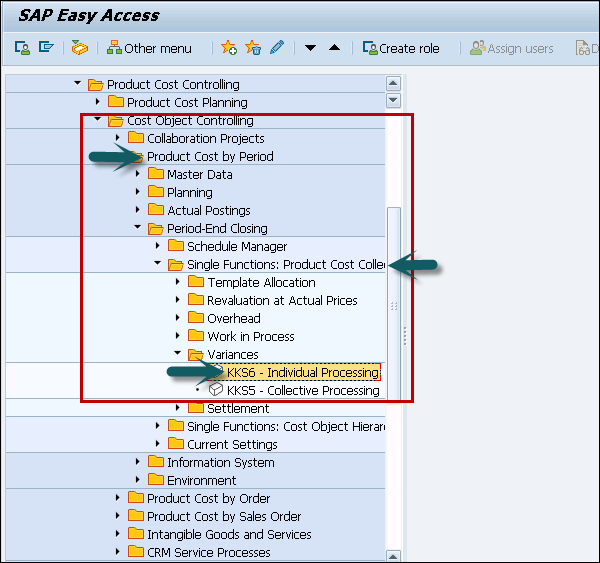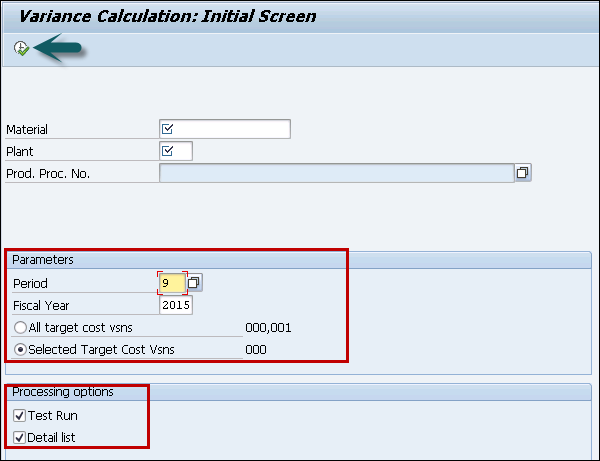# SAP CCA - Variance Calculation

When actual cost on cost center is different from target cost or planning was over/under, you can see a variance in controlling component. Using Variance calculation, you can analyze the actual balance and to find variance of actual cost from target cost with different activity types.

To perform variance calculation, follow the given steps.

Step 1 − Navigate to Accounting → Controlling → Product Cost Controlling → Cost Object Controlling → Product Cost by Period or Product Cost by Order → Period-End Closing → Single Functions: Product Cost Collector → Single Functions: Cost Object Hierarchy → Variances → Individual Processing or Collective Processing.Step 2 − If you want to calculate the variances for a single cost object hierarchy, you have to select the option - Individual Processing and to calculate the variances for all cost object hierarchies in a controlling area, you have to select Collective Processing.

Step 3 − Next, define whether you want to calculate the variances for all target cost versions or only for a particular target cost version.

Step 4 − You can select the option- Selected target cost versions and specify the target cost version under → Extras → Set versions.Step 5 − Set the indicators for processing options. Click the Execute button at the top. The system calculates the variances.

When you select Detail list under Processing options, the system displays Variance Calculation List.